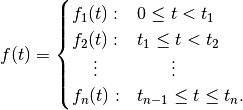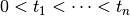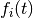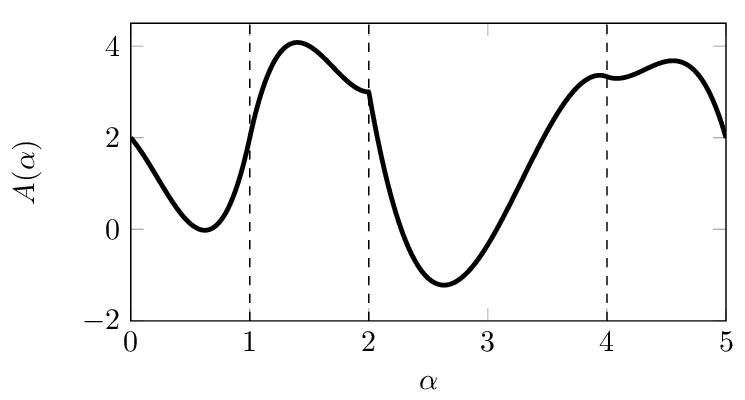# Glossary¶

Piecewise Polynomial

A piecewise function is function of the formwhere. It is naturally possible to define piecewise functions on unbounded domains too, but in typical applications the domain is bounded.

A piecewise polynomial is a piecewise function where each of theis a polynomial, possibly vector-valued. With suitable number of pieces and polynomial degree, piecewise polynomials make a highly expressive function class that is still simple and numerically stable.A piecewise polynomial with four pieces. At the point , this function is continuous but not differentiable. Such a nonsmooth polynomial would not be used for quadrotor trajectory planning.¶

In Crazyswarm, we use degree-7 polynomials with 4-dimensional vector-valued output: (x, y, z) position and yaw angle. The quadrotor’s differential flatness property makes it possible to compute other states (attitude, acceleration, angular velocity) from these four values.

Setpoint

A collection of desired values for some or all of the quadrotor’s state that the feedback controller should try to achieve. For example, a setpoint may specify position, velocity, and acceleration – or just position.